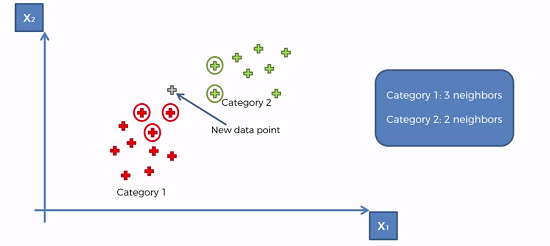## Introduction

Another categorical method of prediction. KNN takes a comparison approach, where new data point is compared to other points that are close by.

It works as shown in the graph below. The Euchlidian distance is calculated between the 5 closest data points. And simply just assign the new data point to the category with largest number of nearest neighbors, in this case it would be category 1.## Steps for KNN

1. Choose the number k of neighbors (default is usually 5)
2. Take the K nearest neighbors of the new data point, accoding to the Euclidean distance (or any other distance formula)
3. Among these K neighbors, count the number of data points in each category
4. Assign the new data point to the category where you counted the most neighbors

## Building the K-NN in Python

Use classsification Template and preprocess the data.

import kNeighborsClassifier from the sklearn.neighbors library and fit the object to the training set.

‘KNeighborsClassifier’ has the following parameters : n_neighbors : # of neighbors to use metric : Distance metric for use for tree. Use ‘minkowski’ p : Power parameter. Euclidean distance = 2.

from sklearn.neighbors import KNeighborsClassifier
classifier = KNeighborsClassifiers(n_neighbors = 5, metric = 'minkowski', p = 2)
classifier.fit(X_train, y_train)